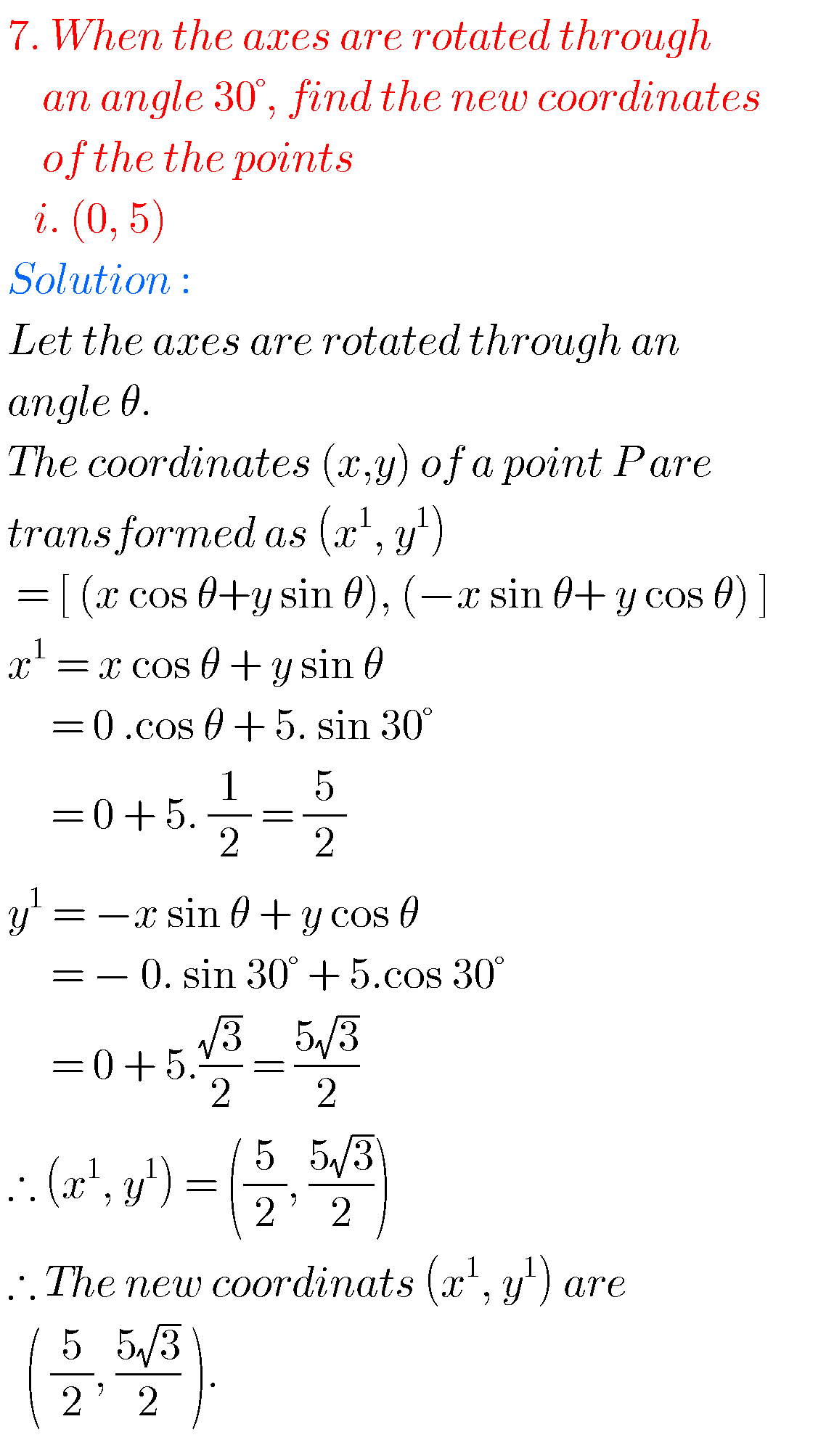## Inter maths 1b solutions

Intermediate maths 1b solutions Inter maths 1B solutions for textbook are given. These solutions are very easy to understand. Study the text book lessons very well. Observe the solutions and try them in your own methods. Intermediate mathematics 1b solutions Inter Maths 1B solutions for textbook Locus Exercise 1(a) Transformation of Axes Exercise 2(a) The …